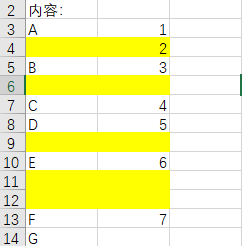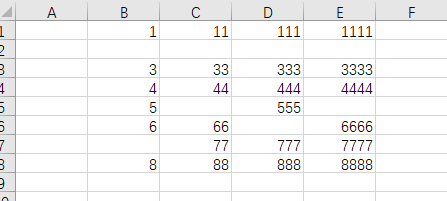• 2021-03-11 22:43:55如题，预计打算把黄色标示的行全部一齐删除。
如果数据比较大达到几十万行的话，循环寻找A列为空则比较低效。
若用SpecialCells函数把空白单元格全部找到而且选中，则不需要循环，速度较快。

Sub Test ()
dim blk, blk2 as range
dim m as Long
Range("A1:A" & lRow).SpecialCells(xlCellTypeBlanks).Select
Set blk = Selection
Set blk2 = Rows(Selection.Row)
For Each m In blk
Set blk2 = Union(blk2, Rows(m.Row))
Next
blk2.Delete
End Sub



谢谢！excel
更多相关内容
• VBA示例函数之 删除空白，供初学者参考，大牛勿进~~~~~~~
• word、wps中使用vba删除所有表格指定 处理word表格搞得人头大，找时间百度一波写了个函数处理标记删除的问题，根据这一套逻辑还可以实现很多功能，供各位参考。 要点： 双循环遍历所有表表头 InStr函数匹配具体...

### word、wps中使用vba删除所有表格指定列

处理word表格搞得人头大，找时间百度一波写了个函数处理标记删除的问题，根据这一套逻辑还可以实现很多功能，供各位参考。

要点：
双循环遍历所有表表头
InStr函数匹配具体内容（word表格多半有特殊字符，相等匹配较难保证）
在内循环结束后进行删除，否则将出错

用法：
1、定义条件，如 InStr(value1, “学号”)，多条件拼接(如 InStr(value1, “学号”)>0 or InStr(value1, “姓名”) >0)
2、新建宏名range，粘贴内容到vba编辑器
3、执行

效果：代码：



'ver 2.0

Sub range()
'遍历文档所有表格，删除表头第一行含有特定字符串的列
Dim value1 As String
Dim target() As Integer
For i = 1 To ActiveDocument.Tables.Count
Dim targetLen As Integer
targetLen = 0
For j = 1 To ActiveDocument.Tables(i).Columns.Count
value1 = ActiveDocument.Tables(i).Columns(j).Cells(1).range.Text
'这里定义条件，可以定义多个条件
If InStr(value1, "测试") > 0 or InStr(value1, "学号") > 0 Then
targetLen = targetLen + 1
ReDim Preserve target(targetLen)
target(targetLen - 1) = j
Else
End If
Next j
If targetLen <> 0 Then
' MsgBox targetLen
' 修正删除操作带来的列号偏移
Dim shift As Integer
shift = 0
For k = 0 To (targetLen - 1)
' MsgBox target(k)
ActiveDocument.Tables(i).Columns(target(k) - shift).Delete
shift = shift + 1
Next k
End If
Next i
MsgBox ("执行完毕。")
End Sub


使用过程中遇到复合表格遍历报错问题，修改加入错误处理跳过
（注意，因需求变动这里已经改成了匹配第一列，如仍需匹配第一行，请按上一段代码循环条件设置）

'ver 3.0
Dim value1 As String
Dim target() As Integer
For i = 1 To ActiveDocument.Tables.Count
'MsgBox ("遍历表 " & i & "。")
Dim targetLen As Integer
targetLen = 0
For j = 1 To ActiveDocument.Tables(i).Rows.Count
On Error GoTo flag
value1 = ActiveDocument.Tables(i).Rows(j).Cells(1).range.Text
'这里定义条件
If InStr(value1, "test1") > 0 Or InStr(value1, "test2") > 0  Then
targetLen = targetLen + 1
ReDim Preserve target(targetLen)
target(targetLen - 1) = j
Else
End If
Next j
If targetLen <> 0 Then
' MsgBox targetLen
' 修正删除操作带来的列号偏移
Dim shift As Integer
shift = 0
For k = 0 To (targetLen - 1)
' MsgBox target(k)
ActiveDocument.Tables(i).Rows(target(k) - shift).Delete
shift = shift + 1
Next k
End If
flag:
Resume continue
continue:
Next i
MsgBox ("执行完毕。")
End Sub


如需删除而非跳过复合行，则删除代码需换成下列实现形式

 With ActiveDocument
.Tables(1).Cell(2, 2).Delete ShiftCells:=wdDeleteCellsEntireRow
End With

展开全文wps openoffice
• Excel中用VBA实现删除空行和空列.docx
• VBA删除如下内容，解决思路都不同 删除1的空行（本文要做的） 删除整个区域内的空行 删除整个区域内的空格（这个一般很少有这种需求，用处不大----可用currentregion .specialcells()解决） 下...

# 1 要解决的问题：删除某列中的空单元格/空行

暂时只实现了删除一列中的空行，并没有实现多行的判断空行和删除方法。----之后再做更复杂的

## 1.1 需求分析

用VBA删除如下内容，解决思路都不同

1. 删除1列的空行（本文要做的）
2. 删除整个区域内的空行
3. 删除整个区域内的空格（这个一般很少有这种需求，用处不大----可用currentregion .specialcells()解决
4. 下面是原始数据，下面看看如何处理# 2 如果是删除全表/某区域的空单元格, 直接在当前列删除

### 简洁代码：删除区域内所有空单元格 cells ---实际需要少，so用处不大

使用 sheet.usedrange / region.currentregion .specialcells()

• 删除，表格页面内使用区域的空格所在的行
• 局限性就是表格的上方，左边还会存在一些空行空列，不过这个手动删下就可以了
• 这个只是删除了空的cells 并不是删除了空行
Sub 删空单元格()

ActiveSheet.UsedRange.SpecialCells(xlCellTypeBlanks).Delete

End Sub

# 3 如果是删一列的空行

## 3.1 代码1：基础代码，假设确认知道这列的长度，关键是倒着删

• 熟悉 isempty() 或者用 if   xxxx=""
• 删除单元格后，要设置属性 shift:=xlup等
• 需要倒着删除，否则会因为一边删除行，一遍重新排列有问题
Sub 删一列的空行()

For i = 20 To 1 Step -1    '需要倒着删除
If IsEmpty(Cells(i, 1)) Then
Cells(i, 1).Delete shift:=xlUp
End If
Next i

End Sub

### 3.2 改进版: 先查这一列的非空最大行数

Sub jackma_delete_row()

For i = Range("c65536").End(xlUp).Rows To 1 Step -1

If IsEmpty(Cells(i, 3)) Then
rows(i).Delete shift:=xlUp
End If

Next i

End Sub

### 3.3 也可以不用 isempty()  函数，直接判断  if cells()=""

• 直接判断 if cells(i,j) =""
• 这种删除整行不合适  rows(i) .delete，会影响其他列的元素
Sub ponyma_del_row1()

For i = Range("c65536").End(xlUp).Row To 1 Step -1
If Cells(i, 3) = "" Then
Cells(i, 3).Delete
'      Rows(i).Delete
End If

Next i

End Sub


3.3 如果是要先删除前面的 重复行，可以正着查询。


'这次收到的需求是，要删掉相同的行里，前面的行
Sub del_test2()

Dim ws1 As Object
Dim ws2 As Object
Dim ws3 As Object

Set ws1 = Worksheets("sheet1")
Set ws2 = Worksheets("sheet2")
Set ws3 = Worksheets("sheet3")

'maxr1 = ws1.Range("a1").End(xlDown).Row

'删行后会导致行数变少
'End(xlDown) 最大的问题还是可能一列中间有空的，断了，查找不准
'反过来查比较准, range("a9999").end(xlup).row

maxr1 = 999       '其实经验发现，把这些配置值放表里出错可能性大，要么弄个控件，要么干脆放代码里

For i = 1 To maxr1

If WorksheetFunction.CountIf(ws1.Columns(1), ws1.Cells(i, 1)) > 1 Then
ws1.Rows(i).Delete
End If

Next

Debug.Print "done"

End Sub

## 4 不动原数据，将非空数据放到另外一列

### 4.1 错误版：

• 要明白“写入列”的循环数，明显和“输出列”的循环数 应该不同！
• 这么写相当于 输出列 那行为空的时候，只是不往 写入列写，所以没覆盖，也是空的
Sub jackma_delete_row2()

For i = 1 To Range("c65536").End(xlUp).Rows

If Not IsEmpty(Cells(i, 3)) Then
Cells(i, 9) = Cells(i, 3)
End If

Next i

End Sub


### 4.2 正确版： 写入列/输出列，应该是单独的循环变量！

Sub jackma_delete_row2()
k = 1

For i = 1 To Range("c65536").End(xlUp).Rows

If Not IsEmpty(Cells(i, 3)) Then
Cells(k, 9) = Cells(i, 3)
k = k + 1
End If

Next i

End Sub


# 5 先写入数组array中，再写到其他地方，据说这样能大幅提高速度！

把需要的筛选的数据，存在数据，然后从数组写到需要的地方，这是个好习惯

### 第1版：局限性很大，没有自动查这列的 元素个数，以及 最大非空行是多少

Sub 删除空格4()
Dim arr1()                          '定义了一个数组，并且是动态数组，因为没指定大小

ReDim arr1(11)                      '动态数组，使用前必须重新redim，数组大小
j = 0                              'j=1开始，不会越界，但arr1(0)为空，因为赋值跳过了它
For i = 1 To 11 Step 1
If Not IsEmpty(Cells(i, 1)) Then
arr1(j) = Cells(i, 1)
j = j + 1
End If
Next i

For j = 0 To UBound(arr1())
Cells(j + 1, 9) = arr1(j)      '单元格得从1开始，arr()，默认得从0开始，但可以改
Next j

End Sub

### 第2版重写

• 注意debug.print用来监测的时候，需要注意，放在循环的位置，尤其是在k=K+1这种变化时，和放在哪个for循环之内外！

Sub ponyma_array22()
Dim arr1()  '当数组定义，且默认开始的index为0！ preserve时需要有0的index
'dim arr1 当变量定义

k = 1
m = 1

ReDim arr1(0 To Application.WorksheetFunction.CountA(Range("c:c")))

For i = 1 To Range("c65536").End(xlUp).Row Step 1
If Cells(i, 3) <> "" Then

Debug.Print Cells(i, 3)
arr1(k) = Cells(i, 3)
Debug.Print arr1(k)
k = k + 1
'          Debug.Print arr1(k),写在这里问题1：k已经变了，下一个k还没赋值为空，2最后的k越界
'          循环是很精巧的，放的地方很讲究，放得不对，就错误百出
'           Debug.Print arr1(k) 如果放在k=K+1 后，就看起来没打印出东西，因为都打印的arr1的空元素

End If
Next i

For j = 1 To UBound(arr1(), 1)
Cells(m, 10) = arr1(j)
m = m + 1
Next j

End Sub

### 第3版

Option Explicit

Sub ponyma1()

Dim arr1()
Dim k1, k2, k
Dim i, j
k1 = WorksheetFunction.CountA(Range("a:a"))
k2 = Range("a65536").End(xlUp).Row
Debug.Print "这列非空数据个数k1=" & k1
Debug.Print "这列最后1个有数据的行数k2=" & k2

'arr1(0) = 1
'ReDim Preserve arr1(1, k)
'这样会越界，因为你需要preserve数据。但是index系不符合
'但是，如果不preserve 就无所谓
'或者虽然 dim arr1() 是动态数据从index0开始，但是arr1()一直为空,preserve也不会出现index越界问题

ReDim Preserve arr1(1 To k1)
'ReDim Preserve arr1(1, k1) 这样就会越界。。。因为语法是2维数组了！
'ReDim Preserve arr1(1 to k1) 这样就对的

k = 1
For i = 1 To k2
If Cells(i, 1) <> "" Then
arr1(k) = Cells(i, 1)
Debug.Print arr1(k)
k = k + 1
End If
Next i

展开全文• sub1.删除工作表所有空行 sub2.删除工作表所有空列 sub3.删除选中单列包含指定字符的行 sub4.删除选中单列不含指定字符的行

## sub1.删除工作表所有空行

Sub 删除工作表所有空行()
Dim first_row, last_row, i
first_row = ActiveSheet.UsedRange.Row
last_row = first_row + ActiveSheet.UsedRange.Rows.count - 1
For i = last_row To first_row Step -1   '倒序循环
If WorksheetFunction.CountA(Rows(i)) = 0 Then
Rows(i).Delete  '删除行
End If
Next
End Sub


## sub2.删除工作表所有空列

Sub 删除工作表所有空列()
Dim first_col, last_col, i
first_col = ActiveSheet.UsedRange.Column
last_col = first_col + ActiveSheet.UsedRange.Columns.count - 1
For i = last_col To first_col Step -1   '倒序循环
If WorksheetFunction.CountA(Columns(i)) = 0 Then
Columns(i).Delete  '删除列
End If
Next
End Sub


## sub3.删除选中单列包含指定字符的行

Sub 删除选中单列包含指定字符的行()
'选中单列整列、单列部分都支持
Dim rng As Range, arr, first_row, last_row, first_col, i, j
'--------------------参数填写：arr，指定条件字符串数组；title_row，表头行数
'要删除的字符串数组，空值为删除空单元格，可使用模式匹配
arr = Array("*一", "*三", "*五")
title_row = 1        '表头行数，不执行删除
Set rng = Intersect(ActiveSheet.UsedRange, Selection)  'intersect语句避免选择整列造成无用计算
If rng.Columns.count > 1 Then Debug.Print "仅支持单列": Exit Sub  '仅支持单列，多列则退出
first_row = WorksheetFunction.Max(title_row, rng.Row)  '表头行与选中区域开始行号的大值
last_row = rng.Row + rng.Rows.count - 1  '选中区域结束行号
first_col = rng.Column  '选中区域开始列号

If rng.Row = 1 Then  '选中单列整列
For i = last_row To title_row + 1 Step -1  '倒序循环
For Each j In arr
'只要有一个符合，就删除
If Cells(i, first_col) Like j Then Rows(i).Delete
Next
Next
ElseIf rng.Row > 1 Then  '选中单列部分
For i = last_row To first_row Step -1  '倒序循环
For Each j In arr
If Cells(i, first_col) Like j Then Rows(i).Delete
Next
Next
End If
End Sub


### 举例

A列选中运行sub3后得到C列效果## sub4.删除选中单列不含指定字符的行

Sub 删除选中单列不含指定字符的行()
'选中单列整列、单列部分都支持
Dim rng As Range, arr, first_row, last_row, first_col, i, j, del_if As Boolean
'--------------------参数填写：arr，指定条件字符串数组；title_row，表头行数
'要保留的字符串数组，空值为保留空单元格，可使用模式匹配
arr = Array("*一", "*三", "*五")
title_row = 1        '表头行数，不执行删除
Set rng = Intersect(ActiveSheet.UsedRange, Selection)  'intersect语句避免选择整列造成无用计算
If rng.Columns.count > 1 Then Debug.Print "仅支持单列": Exit Sub  '仅支持单列，多列则退出
first_row = WorksheetFunction.Max(title_row, rng.Row)  '表头行与选中区域开始行号的大值
last_row = rng.Row + rng.Rows.count - 1  '选中区域结束行号
first_col = rng.Column  '选中区域开始列号

If rng.Row = 1 Then   '选中单列整列
For i = last_row To title_row + 1 Step -1  '倒序循环
del_if = True   '初始为删除
For Each j In arr
'只要有一个符合，就不删除
If Cells(i, first_col) Like j Then del_if = False: Exit For
Next
'都不符合，删除
If del_if Then Rows(i).Delete
Next
ElseIf rng.Row > 1 Then  '选中单列部分
For i = last_row To first_row Step -1  '倒序循环
del_if = True    '初始为删除
For Each j In arr
If Cells(i, first_col) Like j Then del_if = False: Exit For
Next
If del_if Then Rows(i).Delete
Next
End If
End Sub


### 举例

A列选中运行sub4后得到C列效果## sub5.删除选中列重复的整行

对于选中多行多列区域，在一行中所有列的内容都重复，则删除该行，仅保留唯一一行，注意区分字母大小写

Sub 选中列去重()
'适用单/多列选中、单/多列部分选中，去重删除整行
Dim rng As Range, dict As Object, first_row, last_row, first_col, last_col, i, j, res
Set rng = Intersect(ActiveSheet.UsedRange, Selection)  'intersect语句避免选择整列造成无用计算
first_row = rng.Row     '选中区域开始行号
last_row = first_row + rng.Rows.count - 1  '选中区域结束行号
first_col = rng.Column  '选中区域开始列号
last_col = first_col + rng.Columns.count - 1  '选中区域结束列号
Set dict = CreateObject("scripting.dictionary")

For i = last_row To first_row Step -1   '倒序循环，避免遗漏
res = ""
For j = first_col To last_col
res = res & CStr(Cells(i, j).Value)
Next
If Not dict.Exists(res) Then  '字典键不存在，新增
dict(res) = ""
Else
Rows(i).Delete  '删除行
End If
Next

End Sub


### 举例

《excel吧提问-多行多列重复数据筛选》

多列去重前选中A-D列，运行sub5，获得结果展开全文excel
• 今天有网友问，有没有一键删除空白行的代码，下面是一件删除A空白行整行的代码 ，各位请测试后使用！ Sub 一键删除空白行() Columns("A:A").SpecialCells(xlCellTypeBlanks).EntireRow.Delete End Sub ...c# 数据库开发 python
• excel表格处理当前工作表时，如果有几行中穿插着空白行，把下面代码复制进去，自动删除掉 Sub DeleteBlankRow() Dim IngFirstRow As Long Dim IngLastRow As Long Dim a As Integer IngFirstRow = ...
• Sub Del() num = ActiveSheet.UsedRange.Rows.Count() For i = 1 To num If Cells(i, 9) = "" Then Rows(i).Delete End If Next End Sub
• office 365新增函数《TAKE 函数》和《DROP 函数》可以获取/删除单元格区域开头或结尾连续行、，并返回一个单元格区域对于没有office 365又想使用这个函数，就只能自己写VBA代码自定义函数了row和col参数：正数为行...excel
• 在Excel中删除空行和空列的方法有很多，下面的方法是用VBA代码来删除工作表指定区域中的空行和空列：Option Explicit Sub Delete_Empty_Rows() Dim rnArea As Range Dim lnLastRow As Long, i As Long, j As Long...delete excel
• 批量标色表格两列的重复值为不同颜色，以及删除重复项和一
• 实例需求：由于原始数据文件不规范，导入Excel的数据存在空列（例如A列），并且位置不固定，现需要使用代码删除空列数据整理 数据清洗
• 大家好，今天继续讲解VBA数据库解决方案的第14讲：如何动态的在数据库中建立数据表。我们在什么是数据库中讲解讲过，表是一种关系，那么这种关系可否能实现动态的建立呢？也就是说，我们在程序中用代码建立数据表，...
• 今天要说的这段代码呢，同样是因为同事妹子有需求而...那么如果有很多并且每一都要这样做，是不是瞬间觉得不会再爱了→。→ 为了生动形象，我特意做了个表格。就像下图这样，类似红框里这种情况都要去合并单元格。合并
• Sub aa() Dim arr As Variant Dim i As Integer Dim j As Integer Dim rng As Range Dim temp As Variant arr = Range("a1", [a65536].End(3)).Value j = 1 For i ...
• VBA取得EXCEL表格中的行数和数初学EXCEL宏的童鞋，总是很想知道表格中含有数据的行数和数，尤其是行数和数不确定的情况下。这样可以避免很多的错误，并且可以提高效率。但每次用到的时候到网上查找时，总是给...
• 在exce中删除空行和空列的方法有很多，相对而言删除空行较为简单，只需进行筛选，将空白行筛选出来，删除即可，但要删除空列比较困难。因为你不能按列进行筛选删除。Excel中没有这个功能。当然你可以用另外一种方法...
• ridx = 3 '我要清理的内容是从“清理邮箱”工作表的第3行第5开始的，因此ridx起始值为3，下面的所有标也都是5 Do While w.Cells(ridx, 5) <> "" org_email = VBA.Trim(w.Cells(ridx, 5)) '清理内部空格之前先把...经验分享
• columns是当前工作表所有的集合 Columns(1).select 选择第一 Columns.Select 选择所有 上面说到了怎么选择单元格，行，，区域，都是直接指定的，有明确目标的，但我们学习VBA就是要实现智能化，自动化，...
• 目录 示例： 实现代码1： 单元格删除Delete方法 ...现在需要做统计，希望能够将这些空行批量删除，该如何用VBA实现？ 日期/时间 产品 数量 2012/10/29 产品1 83 2012/10/30 产品1 184 ..
• ）, 去空行的，合并的，删除其他的 为了避免EXCEL表过大的效率问题，限制了选择了行数，数，否则很慢，效率很低。带来的问题是需要手动修改行数和数参数。对不会改VBA代码的人是个问题。 没有界面化，按钮化 ...
• 确保在选择中包括要删除的最左边一左侧的和要删除的最右边一右侧的。 Again, click “Find & Select” in the “Editing” section of the “Home” tab and select “Go To Special…” from the drop-...excel java 开发工具 项目管理
• Dim r% Do r = r+2 '原始r=0,然后r=0+2=2 Rows(r).Insert '在第2行进行插入，这时候原始第1行和第2行中间就有了空行 Loop Util Cells(r+1,1) = "" '执行这个循环直到 r 行1的下方单元格为 End Sub **********...
• 问题 左上角为a1 比如其中，key2 ,key4, key6 后面都可能带空格 key1 key2 key3 key4 key5 key6 key7 key8 key9 key10 key11 key12 key10 key10 ...VBA方法 所有的工...
• excel宏命令删除列 删除Excel宏安全警告 (Remove Excel Macros Security Warning)Even though an Excel workbook doesn't contain any macros, sometimes when you open a file, a security warning appears. ...python java linux excel 数据库
• 要使用VBA，首先打开你的Excel电子表格，然后将Microsoft Visual Basic窗口打开，这样才能使用VBA代码！ 　方法是：在Excel中直接按下组合键Alt F11即可打开VB窗口，倘若VB...删除第8个单元格为的行，范围从1行......